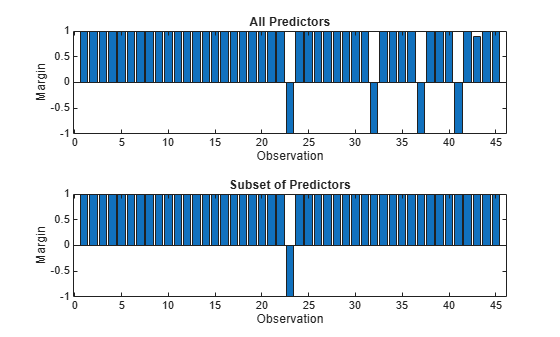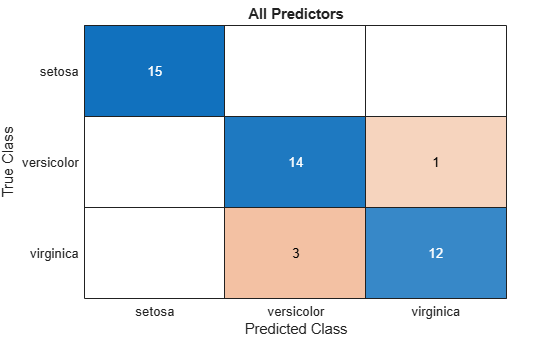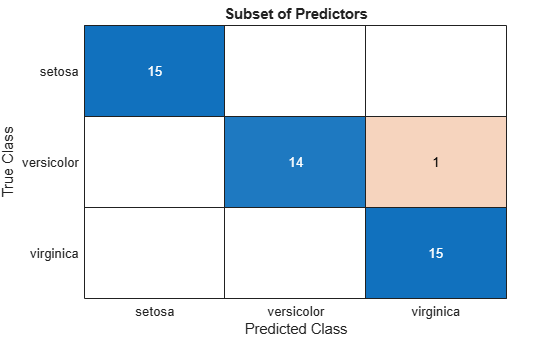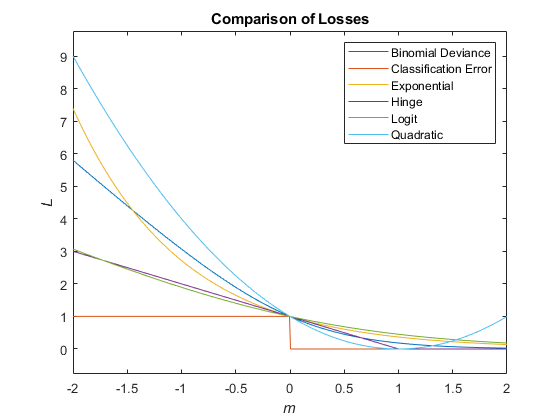# loss

Classification loss for neural network classifier

## Syntax

``L = loss(Mdl,Tbl,ResponseVarName)``
``L = loss(Mdl,Tbl,Y)``
``L = loss(Mdl,X,Y)``
``L = loss(___,Name,Value)``

## Description

example

````L = loss(Mdl,Tbl,ResponseVarName)` returns the classification loss for the trained neural network classifier `Mdl` using the predictor data in table `Tbl` and the class labels in the `ResponseVarName` table variable.`L` is returned as a scalar value that represents the classification error by default.```
````L = loss(Mdl,Tbl,Y)` returns the classification loss for the classifier `Mdl` using the predictor data in table `Tbl` and the class labels in vector `Y`.```
````L = loss(Mdl,X,Y)` returns the classification loss for the trained neural network classifier `Mdl` using the predictor data `X` and the corresponding class labels in `Y`.```
````L = loss(___,Name,Value)` specifies options using one or more name-value arguments in addition to any of the input argument combinations in previous syntaxes. For example, you can specify that columns in the predictor data correspond to observations, specify the loss function, or supply observation weights.```

## Examples

collapse all

Calculate the test set classification error of a neural network classifier.

Load the `patients` data set. Create a table from the data set. Each row corresponds to one patient, and each column corresponds to a diagnostic variable. Use the `Smoker` variable as the response variable, and the rest of the variables as predictors.

```load patients tbl = table(Diastolic,Systolic,Gender,Height,Weight,Age,Smoker);```

Separate the data into a training set `tblTrain` and a test set `tblTest` by using a stratified holdout partition. The software reserves approximately 30% of the observations for the test data set and uses the rest of the observations for the training data set.

```rng("default") % For reproducibility of the partition c = cvpartition(tbl.Smoker,"Holdout",0.30); trainingIndices = training(c); testIndices = test(c); tblTrain = tbl(trainingIndices,:); tblTest = tbl(testIndices,:);```

Train a neural network classifier using the training set. Specify the `Smoker` column of `tblTrain` as the response variable. Specify to standardize the numeric predictors.

```Mdl = fitcnet(tblTrain,"Smoker", ... "Standardize",true);```

Calculate the test set classification error. Classification error is the default loss type for neural network classifiers.

`testError = loss(Mdl,tblTest,"Smoker")`
```testError = 0.0671 ```
`testAccuracy = 1 - testError`
```testAccuracy = 0.9329 ```

The neural network model correctly classifies approximately 93% of the test set observations.

Perform feature selection by comparing test set classification margins, edges, errors, and predictions. Compare the test set metrics for a model trained using all the predictors to the test set metrics for a model trained using only a subset of the predictors.

Load the sample file `fisheriris.csv`, which contains iris data including sepal length, sepal width, petal length, petal width, and species type. Read the file into a table.

`fishertable = readtable('fisheriris.csv');`

Separate the data into a training set `trainTbl` and a test set `testTbl` by using a stratified holdout partition. The software reserves approximately 30% of the observations for the test data set and uses the rest of the observations for the training data set.

```rng("default") c = cvpartition(fishertable.Species,"Holdout",0.3); trainTbl = fishertable(training(c),:); testTbl = fishertable(test(c),:);```

Train one neural network classifier using all the predictors in the training set, and train another classifier using all the predictors except `PetalWidth`. For both models, specify `Species` as the response variable, and standardize the predictors.

```allMdl = fitcnet(trainTbl,"Species","Standardize",true); subsetMdl = fitcnet(trainTbl,"Species ~ SepalLength + SepalWidth + PetalLength", ... "Standardize",true);```

Calculate the test set classification margins for the two models. Because the test set includes only 45 observations, display the margins using bar graphs.

For each observation, the classification margin is the difference between the classification score for the true class and the maximal score for the false classes. Because neural network classifiers return classification scores that are posterior probabilities, margin values close to 1 indicate confident classifications and negative margin values indicate misclassifications.

```tiledlayout(2,1) % Top axes ax1 = nexttile; allMargins = margin(allMdl,testTbl); bar(ax1,allMargins) xlabel(ax1,"Observation") ylabel(ax1,"Margin") title(ax1,"All Predictors") % Bottom axes ax2 = nexttile; subsetMargins = margin(subsetMdl,testTbl); bar(ax2,subsetMargins) xlabel(ax2,"Observation") ylabel(ax2,"Margin") title(ax2,"Subset of Predictors")```Compare the test set classification edge, or mean of the classification margins, of the two models.

`allEdge = edge(allMdl,testTbl)`
```allEdge = 0.8198 ```
`subsetEdge = edge(subsetMdl,testTbl)`
```subsetEdge = 0.9556 ```

Based on the test set classification margins and edges, the model trained on a subset of the predictors seems to outperform the model trained on all the predictors.

Compare the test set classification error of the two models.

```allError = loss(allMdl,testTbl); allAccuracy = 1-allError```
```allAccuracy = 0.9111 ```
```subsetError = loss(subsetMdl,testTbl); subsetAccuracy = 1-subsetError```
```subsetAccuracy = 0.9778 ```

Again, the model trained using only a subset of the predictors seems to perform better than the model trained using all the predictors.

Visualize the test set classification results using confusion matrices.

```allLabels = predict(allMdl,testTbl); figure confusionchart(testTbl.Species,allLabels) title("All Predictors")``````subsetLabels = predict(subsetMdl,testTbl); figure confusionchart(testTbl.Species,subsetLabels) title("Subset of Predictors")```The model trained using all the predictors misclassifies four of the test set observations. The model trained using a subset of the predictors misclassifies only one of the test set observations.

Given the test set performance of the two models, consider using the model trained using all the predictors except `PetalWidth`.

## Input Arguments

collapse all

Trained neural network classifier, specified as a `ClassificationNeuralNetwork` model object or `CompactClassificationNeuralNetwork` model object returned by `fitcnet` or `compact`, respectively.

Sample data, specified as a table. Each row of `Tbl` corresponds to one observation, and each column corresponds to one predictor variable. Optionally, `Tbl` can contain an additional column for the response variable. `Tbl` must contain all of the predictors used to train `Mdl`. Multicolumn variables and cell arrays other than cell arrays of character vectors are not allowed.

• If `Tbl` contains the response variable used to train `Mdl`, then you do not need to specify `ResponseVarName` or `Y`.

• If you trained `Mdl` using sample data contained in a table, then the input data for `loss` must also be in a table.

• If you set `'Standardize',true` in `fitcnet` when training `Mdl`, then the software standardizes the numeric columns of the predictor data using the corresponding means and standard deviations.

Data Types: `table`

Response variable name, specified as the name of a variable in `Tbl`. If `Tbl` contains the response variable used to train `Mdl`, then you do not need to specify `ResponseVarName`.

If you specify `ResponseVarName`, then you must specify it as a character vector or string scalar. For example, if the response variable is stored as `Tbl.Y`, then specify `ResponseVarName` as `'Y'`. Otherwise, the software treats all columns of `Tbl`, including `Tbl.Y`, as predictors.

The response variable must be a categorical, character, or string array; a logical or numeric vector; or a cell array of character vectors. If the response variable is a character array, then each element must correspond to one row of the array.

Data Types: `char` | `string`

Class labels, specified as a categorical, character, or string array; logical or numeric vector; or cell array of character vectors.

• The data type of `Y` must be the same as the data type of `Mdl.ClassNames`. (The software treats string arrays as cell arrays of character vectors.)

• The distinct classes in `Y` must be a subset of `Mdl.ClassNames`.

• If `Y` is a character array, then each element must correspond to one row of the array.

• The length of `Y` must be equal to the number of observations in `X` or `Tbl`.

Data Types: `categorical` | `char` | `string` | `logical` | `single` | `double` | `cell`

Predictor data, specified as a numeric matrix. By default, `loss` assumes that each row of `X` corresponds to one observation, and each column corresponds to one predictor variable.

Note

If you orient your predictor matrix so that observations correspond to columns and specify `'ObservationsIn','columns'`, then you might experience a significant reduction in computation time.

The length of `Y` and the number of observations in `X` must be equal.

If you set `'Standardize',true` in `fitcnet` when training `Mdl`, then the software standardizes the numeric columns of the predictor data using the corresponding means and standard deviations.

Data Types: `single` | `double`

### Name-Value Arguments

Specify optional comma-separated pairs of `Name,Value` arguments. `Name` is the argument name and `Value` is the corresponding value. `Name` must appear inside quotes. You can specify several name and value pair arguments in any order as `Name1,Value1,...,NameN,ValueN`.

Example: `loss(Mdl,Tbl,"Response","LossFun","crossentropy")` specifies to compute the cross-entropy loss for the model `Mdl`.

Loss function, specified as a built-in loss function name or a function handle.

• This table lists the available loss functions. Specify one using its corresponding character vector or string scalar.

ValueDescription
`'binodeviance'`Binomial deviance
`'classiferror'`Misclassified rate in decimal
`'crossentropy'`Cross-entropy loss (for neural networks only)
`'exponential'`Exponential loss
`'hinge'`Hinge loss
`'logit'`Logistic loss
`'mincost'`Minimal expected misclassification cost (for classification scores that are posterior probabilities)
`'quadratic'`Quadratic loss

For more details on loss functions, see Classification Loss.

• To specify a custom loss function, use function handle notation. The function must have this form:

``lossvalue = lossfun(C,S,W,Cost)``

• The output argument `lossvalue` is a scalar.

• You specify the function name (`lossfun`).

• `C` is an `n`-by-`K` logical matrix with rows indicating the class to which the corresponding observation belongs. `n` is the number of observations in `Tbl` or `X`, and `K` is the number of distinct classes (`numel(Mdl.ClassNames)`. The column order corresponds to the class order in `Mdl.ClassNames`. Create `C` by setting ```C(p,q) = 1```, if observation `p` is in class `q`, for each row. Set all other elements of row `p` to `0`.

• `S` is an `n`-by-`K` numeric matrix of classification scores. The column order corresponds to the class order in `Mdl.ClassNames`. `S` is a matrix of classification scores, similar to the output of `predict`.

• `W` is an `n`-by-1 numeric vector of observation weights.

• `Cost` is a `K`-by-`K` numeric matrix of misclassification costs. For example, ```Cost = ones(K) – eye(K)``` specifies a cost of `0` for correct classification and `1` for misclassification.

Example: `'LossFun','crossentropy'`

Data Types: `char` | `string` | `function_handle`

Predictor data observation dimension, specified as `'rows'` or `'columns'`.

Note

If you orient your predictor matrix so that observations correspond to columns and specify `'ObservationsIn','columns'`, then you might experience a significant reduction in computation time. You cannot specify `'ObservationsIn','columns'` for predictor data in a table.

Data Types: `char` | `string`

Observation weights, specified as a nonnegative numeric vector or the name of a variable in `Tbl`. The software weights each observation in `X` or `Tbl` with the corresponding value in `Weights`. The length of `Weights` must equal the number of observations in `X` or `Tbl`.

If you specify the input data as a table `Tbl`, then `Weights` can be the name of a variable in `Tbl` that contains a numeric vector. In this case, you must specify `Weights` as a character vector or string scalar. For example, if the weights vector `W` is stored as `Tbl.W`, then specify it as `'W'`.

By default, `Weights` is `ones(n,1)`, where `n` is the number of observations in `X` or `Tbl`.

If you supply weights, then `loss` computes the weighted classification loss and normalizes weights to sum to the value of the prior probability in the respective class.

Data Types: `single` | `double` | `char` | `string`

collapse all

### Classification Loss

Classification loss functions measure the predictive inaccuracy of classification models. When you compare the same type of loss among many models, a lower loss indicates a better predictive model.

Consider the following scenario.

• L is the weighted average classification loss.

• n is the sample size.

• For binary classification:

• yj is the observed class label. The software codes it as –1 or 1, indicating the negative or positive class (or the first or second class in the `ClassNames` property), respectively.

• f(Xj) is the positive-class classification score for observation (row) j of the predictor data X.

• mj = yjf(Xj) is the classification score for classifying observation j into the class corresponding to yj. Positive values of mj indicate correct classification and do not contribute much to the average loss. Negative values of mj indicate incorrect classification and contribute significantly to the average loss.

• For algorithms that support multiclass classification (that is, K ≥ 3):

• yj* is a vector of K – 1 zeros, with 1 in the position corresponding to the true, observed class yj. For example, if the true class of the second observation is the third class and K = 4, then y2* = [0 0 1 0]′. The order of the classes corresponds to the order in the `ClassNames` property of the input model.

• f(Xj) is the length K vector of class scores for observation j of the predictor data X. The order of the scores corresponds to the order of the classes in the `ClassNames` property of the input model.

• mj = yj*f(Xj). Therefore, mj is the scalar classification score that the model predicts for the true, observed class.

• The weight for observation j is wj. The software normalizes the observation weights so that they sum to the corresponding prior class probability. The software also normalizes the prior probabilities so they sum to 1. Therefore,

`$\sum _{j=1}^{n}{w}_{j}=1.$`

Given this scenario, the following table describes the supported loss functions that you can specify by using the `'LossFun'` name-value pair argument.

Loss FunctionValue of `LossFun`Equation
Binomial deviance`'binodeviance'`$L=\sum _{j=1}^{n}{w}_{j}\mathrm{log}\left\{1+\mathrm{exp}\left[-2{m}_{j}\right]\right\}.$
Misclassified rate in decimal`'classiferror'`

$L=\sum _{j=1}^{n}{w}_{j}I\left\{{\stackrel{^}{y}}_{j}\ne {y}_{j}\right\}.$

${\stackrel{^}{y}}_{j}$ is the class label corresponding to the class with the maximal score. I{·} is the indicator function.

Cross-entropy loss`'crossentropy'`

`'crossentropy'` is appropriate only for neural network models.

The weighted cross-entropy loss is

`$L=-\sum _{j=1}^{n}\frac{{\stackrel{˜}{w}}_{j}\mathrm{log}\left({m}_{j}\right)}{Kn},$`

where the weights ${\stackrel{˜}{w}}_{j}$ are normalized to sum to n instead of 1.

Exponential loss`'exponential'`$L=\sum _{j=1}^{n}{w}_{j}\mathrm{exp}\left(-{m}_{j}\right).$
Hinge loss`'hinge'`$L=\sum _{j=1}^{n}{w}_{j}\mathrm{max}\left\{0,1-{m}_{j}\right\}.$
Logit loss`'logit'`$L=\sum _{j=1}^{n}{w}_{j}\mathrm{log}\left(1+\mathrm{exp}\left(-{m}_{j}\right)\right).$
Minimal expected misclassification cost`'mincost'`

`'mincost'` is appropriate only if classification scores are posterior probabilities.

The software computes the weighted minimal expected classification cost using this procedure for observations j = 1,...,n.

1. Estimate the expected misclassification cost of classifying the observation Xj into the class k:

`${\gamma }_{jk}={\left(f{\left({X}_{j}\right)}^{\prime }C\right)}_{k}.$`

f(Xj) is the column vector of class posterior probabilities for binary and multiclass classification for the observation Xj. C is the cost matrix stored in the `Cost` property of the model.

2. For observation j, predict the class label corresponding to the minimal expected misclassification cost:

`${\stackrel{^}{y}}_{j}=\underset{k=1,...,K}{\text{argmin}}{\gamma }_{jk}.$`

3. Using C, identify the cost incurred (cj) for making the prediction.

The weighted average of the minimal expected misclassification cost loss is

`$L=\sum _{j=1}^{n}{w}_{j}{c}_{j}.$`

If you use the default cost matrix (whose element value is 0 for correct classification and 1 for incorrect classification), then the `'mincost'` loss is equivalent to the `'classiferror'` loss.

Quadratic loss`'quadratic'`$L=\sum _{j=1}^{n}{w}_{j}{\left(1-{m}_{j}\right)}^{2}.$

This figure compares the loss functions (except `'crossentropy'` and `'mincost'`) over the score m for one observation. Some functions are normalized to pass through the point (0,1).